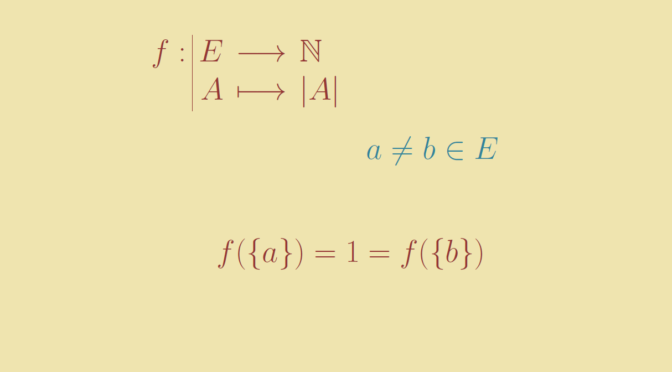A strictly increasing map that is not one-to-one

Consider two partially ordered sets $$(E,\le)$$ and $$(F,\le)$$ and a strictly increasing map $$f : E \to F$$. If the order $$(E,\le)$$ is total, then $$f$$ is one-to-one. Indeed for distinct elements $$x,y \in E$$, we have either $$x < y$$ or $$y < x$$ and consequently $$f(x) < f(y)$$ or $$f(y) < f(x)$$. Therefore $$f(x)$$ and $$f(y)$$ are different. This is not true anymore for a partial order $$(E,\le)$$. We give a counterexample.

Consider a finite set $$E$$ having at least two elements and partially ordered by the inclusion. Let $$f$$ be the map defined on the powerset $$\wp(E)$$ that maps $$A \subseteq E$$ to its cardinal $$\vert A \vert$$. $$f$$ is obviously strictly increasing. However $$f$$ is not one-to-one as for distincts elements $$a,b \in E$$ we have $f(\{a\}) = 1 = f(\{b\})$